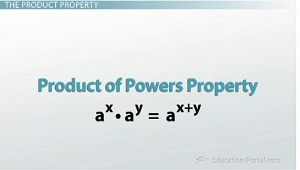# Logarithmic Properties

An error occurred trying to load this video.

Try refreshing the page, or contact customer support.

Coming up next: Practice Problems for Logarithmic Properties

### You're on a roll. Keep up the good work!

Replay
Your next lesson will play in 10 seconds
• 0:11 Logarithmic Properties
• 0:34 The Product Property
• 2:18 The Quotient Property
• 2:38 The Power Property
• 4:35 Lesson Summary

Want to watch this again later?

Timeline
Autoplay
Autoplay
Speed

#### Recommended Lessons and Courses for You

Lesson Transcript
Instructor: Eric Garneau
Along with the change of base property, there are three other logarithmic properties that allow you to manipulate expressions to your advantage. Learn about the product rule, quotient rule and power rule here.

## Logarithmic Properties

Once you've mastered evaluating logs, it's time to learn the tricks. Similar to how multiplication has the distributive property, logarithms have their own properties that will help us down the road when we are doing real-world problems. Because this video is about the nuts and bolts behind the fun stuff, it won't be the most exciting video ever, but I will try to make it as painless as possible.

## The Product Property

The first property we'll learn is called the product property. Because logs are related to exponents, this is, actually, very similar to the exponent property called the product of powers property. It basically tells us that when we multiply two powers we get to add their exponents. As long as you remember that, we can come up with the product property for logs in the following way. Now, what I'm about to do is a mathematical proof of the product property. I'm not going to do this for all the properties in this video, but I do think it's good to see at least one of them so that you know that these things aren't just made up; they actually come from some math.Now, all proofs need to start with some assumed information, so for this one we'll start by saying let log base b of x equal m and log base b of y equal n. That's two equations in logarithmic form, but if I change those same equations to exponential form, I get bm = x and bn = y. Therefore, if I multiply x and y, all I'm really doing is multiplying bm * bn, which then allows us to use the product of powers property of exponents, like we mentioned before, and add the exponents, so I get b(m+n). This is still in exponential form, but if I change it back to logarithmic form, I'd have the log base b of x * y = m + n. But then going back to our original definition of what m and n were and substituting those in this equation, that gives us that the log base b of x * y equals the log base b of x plus the log base b of y. This is exactly what the product property of logarithms is.

## The Quotient & Power Properties

If we do the exact same proof but with division instead of multiplication, we end up using the exponent property called the quotient of powers, and we get the quotient property of logarithms, which is that the log base b of x divided by y is equal to the log base b of x minus the log base b of y. Finally, if we use an exponent in our proof, we end up with the power property of logarithms, which tells us that the log base b of xy is equal to y times log base b of x.

To unlock this lesson you must be a Study.com Member.

### Register to view this lesson

Are you a student or a teacher?

### Unlock Your Education

#### See for yourself why 30 million people use Study.com

##### Become a Study.com member and start learning now.
Back
What teachers are saying about Study.com

### Earning College Credit

Did you know… We have over 160 college courses that prepare you to earn credit by exam that is accepted by over 1,500 colleges and universities. You can test out of the first two years of college and save thousands off your degree. Anyone can earn credit-by-exam regardless of age or education level.